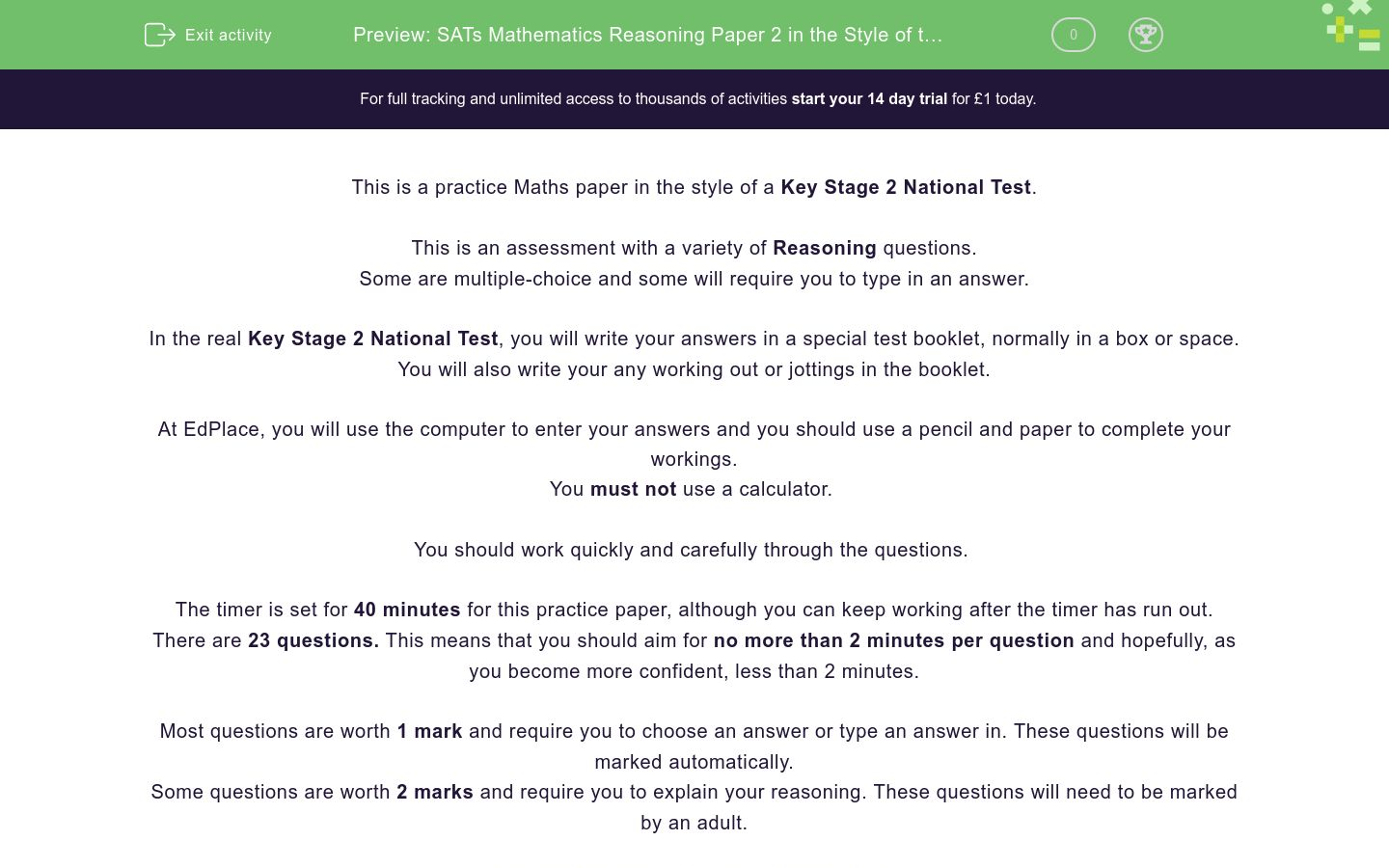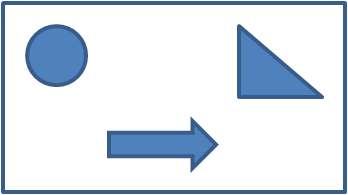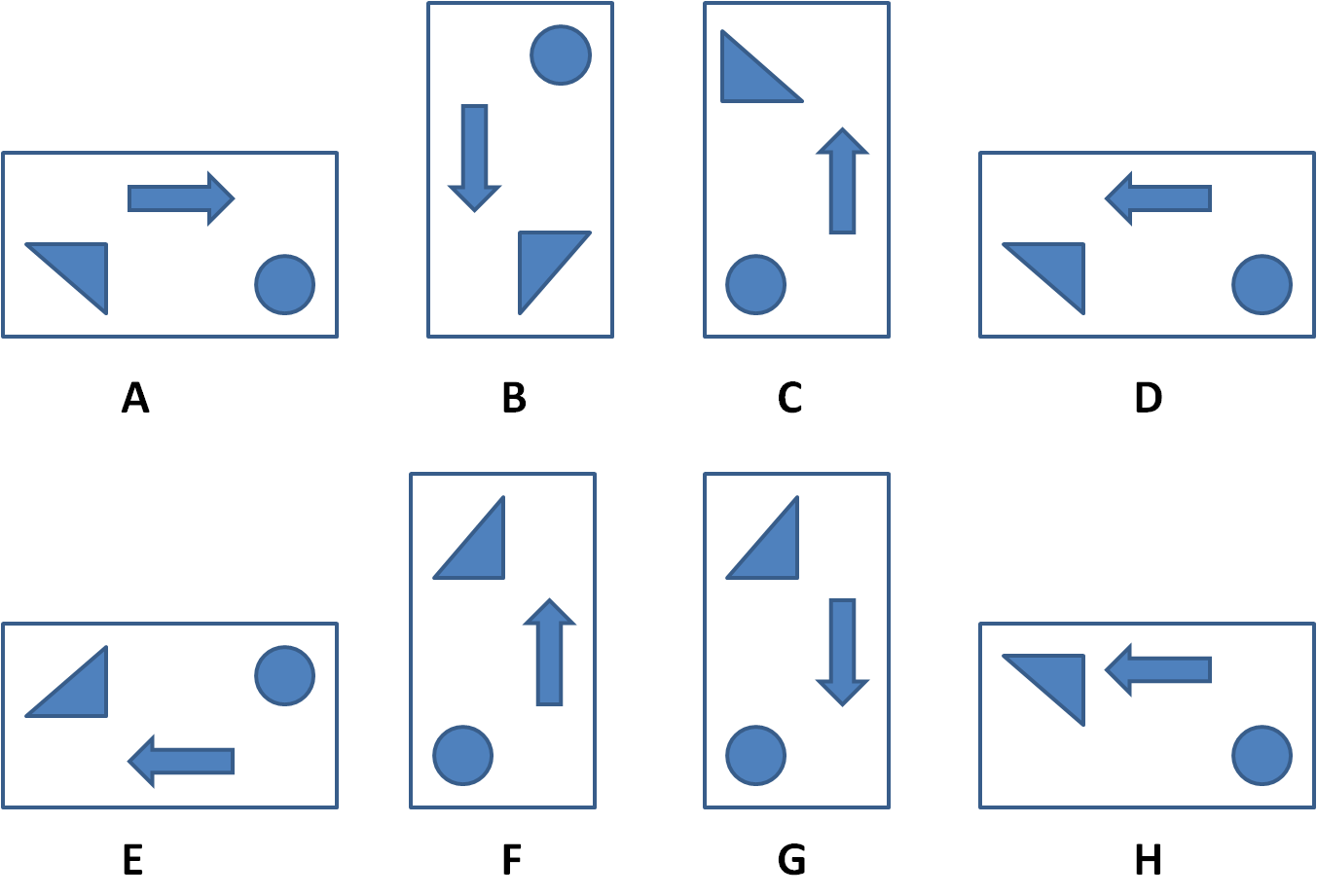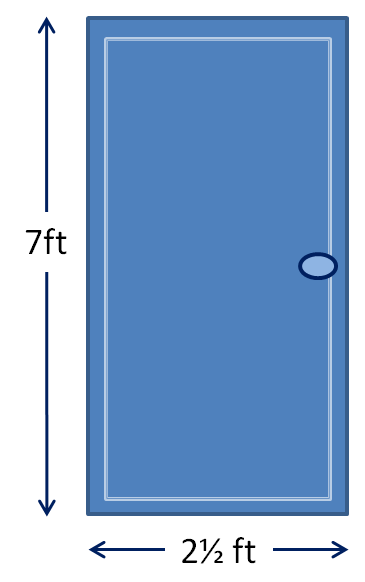# SATs Mathematics Reasoning Paper 2 in the Style of the Key Stage 2 National Tests (Practice 2)

In this assessment, students will be able to complete a timed Reasoning paper in the style of Key Stage 2 SATs.Key stage:  KS 2

Curriculum topic:   SATs Practice Papers

Curriculum subtopic:   Reasoning Practice Papers

Difficulty level:### QUESTION 1 of 10

This is a practice Maths paper in the style of a Key Stage 2 National Test.

This is an assessment with a variety of Reasoning questions.

Some are multiple-choice and some will require you to type in an answer.

In the real Key Stage 2 National Test, you will write your answers in a special test booklet, normally in a box or space.

You will also write your any working out or jottings in the booklet.

At EdPlace, you will use the computer to enter your answers and you should use a pencil and paper to complete your workings.

You must not use a calculator.

You should work quickly and carefully through the questions.

The timer is set for 40 minutes for this practice paper, although you can keep working after the timer has run out.

There are 23 questions. This means that you should aim for no more than 2 minutes per question and hopefully, as you become more confident, less than 2 minutes.

Most questions are worth 1 mark and require you to choose an answer or type an answer in. These questions will be marked automatically.

Some questions are worth 2 marks and require you to explain your reasoning. These questions will need to be marked by an adult.

You may find some of the questions difficult.

If you are struggling to answer a question do not waste time on it, but move onto the next question.

Disclaimer:

We have no affiliation to the Standards and Testing Agency (STA) and these questions represent our own unique content developed by EdPlace Key Stage 2 Maths authors.

None of the content displayed here has been supplied by the STA or any other third party suppliers.

Find the missing numbers in this multiplication grid.## Column B

A
6
B
32
C
36
D
9

Jack has these 10 coins in his pocket.He buys two sweets costing 26p each.

He wishes to use as many coins as possible.

He pays the exact amount.

How many coins does he use to pay for the sweets?

Select the number which is closest to 500.

552

442

449

50

450

Select the calculation which has the greatest answer.

150 ÷ 10

64 ÷ 4

60 ÷ 4

98 ÷ 7

65 ÷ 5

Look at this design.Select all the designs below which are the same as the original above after they have been rotated.A

B

C

D

E

F

G

H

The numbers in this sequence go up 4 each time.

4    8    12    16    ...

The numbers in this sequence go up 5 each time.

5    10    15    20    ...

What is the first number greater than 50 which will be in both sequences?

Find the missing number in the calculation below:

370 ÷ ? + 12 = 49

Look at this number line.

To what number is the red arrow pointing?Here is a function machine.What is the missing number, shown with a ? mark, in this function machine?Antonio thinks of a number.

He adds 5½ to it and then divides the result by 2.

What number did Antonio first think of?

Select each multiplication which has an answer greater than 150.

12 × 2 × 10

2 × 12 × 3

15 × 10

16 × 5 × 2

16 × 2 × 10

4 × 12 × 3One tennis ball costs £1.45.A sealed pack of 4 tennis balls costs £5.

Jack wants to buy 8 tennis balls.

How much does he save by buying 2 packs instead of 8 individual balls?

Show your answer with a decimal point to separate pounds and pence.

12 × 2 × 10

2 × 12 × 3

15 × 10

16 × 5 × 2

16 × 2 × 10

4 × 12 × 3

Place these in order of size, starting with the smallest.

## Column B

1 (smallest)
0.4
2
¼
3
0.14
4 (largest)
41%

Look at this rectangle, made of smaller squares.What percentage of the whole rectangle has been shaded red?

## Column B

1 (smallest)
0.4
2
¼
3
0.14
4 (largest)
41%

Match the calculations which are equal in value.

## Column B

2 x 6
132 ÷ 11
2 x 16
5 x 16
8 x 10
3² × 4
9 x 4
8 x 4

Select all the numbers which give 70 when rounded to the nearest 10.

10

65

70

74

75

77

Which is greater?

10% of 380

20% of 200

25% of 120

15% of 300

Look at this door.On foot (ft) is equivalent to 12 inches.

What is the perimeter of this door?  Give your answer in inches.

A shaded shape is shown on a 1 cm square grid.John cuts outs 4 shapes identical to this and joins them together along the slanted sides to make a cross shape.

What will be the area and perimeter of the new shape?

Aisha says:

"All numbers that end in a 0 are multiples of 5."

Bill says:

"All multiples of 5 end in a 0."

Who is correct?

Ben has some sweets in a jar.

On Monday he eats 20% of the sweets.

On Tuesday he eats 2/5 of the sweets.

There are 16 sweets left.

Look at this pie chart.What fraction of the whole pie chart is the Apple section?

This diagram shows a shape made with a regular hexagon and an equilateral triangle.Sarah thinks the perimeter of the whole shape is 49.5 cm.

Sarah is not correct.

Explain how she got this answer.

What is the actual perimeter?

• Question 1

Find the missing numbers in this multiplication grid.## Column B

A
9
B
6
C
36
D
32
EDDIE SAYS
The missing numbers are: A = 9 B = 6 C = 36 D = 32 Use your knowledge of times tables facts and division facts to work these ones out.
• Question 2

Jack has these 10 coins in his pocket.He buys two sweets costing 26p each.

He wishes to use as many coins as possible.

He pays the exact amount.

How many coins does he use to pay for the sweets?

8
EDDIE SAYS
The answer is 8. Jack buys 2 sweets costing 26p each, which makes a total of 52p. Jack uses two 1p coins, two 5p coins, four 10p coins to make a total of 52p.
• Question 3

Select the number which is closest to 500.

450
EDDIE SAYS
The answer is 450. 552 is 52 away from 500. 442 is 58 away from 500. 449 is 51 away from 500. 50 is 450 away from 500. 450 is just 50 away from 500 so this is the closest.
• Question 4

Select the calculation which has the greatest answer.

64 ÷ 4
EDDIE SAYS
The answer is 64 ÷ 4 as it gives the greatest answer. 150 ÷ 10 = 15 64 ÷ 4 = 16 60 ÷ 4 = 15 98 ÷ 7 = 14 65 ÷ 5 = 13
• Question 5

Look at this design.Select all the designs below which are the same as the original above after they have been rotated.B
D
F
EDDIE SAYS
The answers are B, D and F. A: 180° rotation but arrow points in wrong direction.
B: 90° clockwise rotation.
C: 90° anticlockwise rotation but triangle is wrong.
D: 180° rotation.
E: reflection so can't be rotation.
F: 90° anticlockwise rotation.
G: 90° anticlockwise rotation but arrow is wrong.
H: 180° rotation but triangle is wrong.
• Question 6

The numbers in this sequence go up 4 each time.

4    8    12    16    ...

The numbers in this sequence go up 5 each time.

5    10    15    20    ...

What is the first number greater than 50 which will be in both sequences?

60
EDDIE SAYS
The answer is 60. To solve this, write down the multiples above 50 of 4, then 5: Multiples of 4 greater than 50 are 52, 56, 60, 64, etc. Multiples of 5 greater than 50 are 55, 60, 65, etc. 60 is the first number that appears in both sequences.
• Question 7

Find the missing number in the calculation below:

370 ÷ ? + 12 = 49

EDDIE SAYS
The missing number is 10. Firstly, find the difference between 49 and 12. 49 - 12 = 37 Next, work out the missing number. 370 ÷ 37 = 10 So we know that 370 ÷ 10 = 37
• Question 8

Look at this number line.

To what number is the red arrow pointing?0.8
EDDIE SAYS
The arrow is pointing at 0.8. There are ten divisions between zero and one. So each division on this scale is worth one-tenth. We can count along in steps of 0.1 from zero to reach 0.8.
• Question 9

Here is a function machine.What is the missing number, shown with a ? mark, in this function machine?12
EDDIE SAYS
The missing number is 12. Work backwards to find the missing number. 45 ÷ 9 = 5 5 + 7 = 12
• Question 10

Antonio thinks of a number.

He adds 5½ to it and then divides the result by 2.

What number did Antonio first think of?

14.5
EDDIE SAYS
Antonio stared with the number 14.5. Work backwards, starting with 10 and use inverse operations to solve this problem. 10 × 2 = 20 20 - 5.5 = 14.5
• Question 11

Select each multiplication which has an answer greater than 150.

12 × 2 × 10
16 × 5 × 2
16 × 2 × 10
EDDIE SAYS
The correct answers are: 12 × 2 × 10 = 240 16 × 5 × 2 = 160 16 × 2 × 10 = 320 The answers which are not greater than 150 are: 2 × 12 × 3 = 72 15 × 10 = 150 (which is equal to but not greater than 150) 4 × 12 × 3 = 144
• Question 12One tennis ball costs £1.45.A sealed pack of 4 tennis balls costs £5.

Jack wants to buy 8 tennis balls.

How much does he save by buying 2 packs instead of 8 individual balls?

Show your answer with a decimal point to separate pounds and pence.

EDDIE SAYS
Jack saves £1.60 8 individual balls cost 8 × 1.45 = £11.60 2 packs cost 2 × 5 = £10.00 11.60 - 10.00 = 1.60
• Question 13

Place these in order of size, starting with the smallest.

## Column B

1 (smallest)
0.14
2
¼
3
0.4
4 (largest)
41%
EDDIE SAYS
The correct order is as follows: 0.14 ¼ = 0.25 0.4 = 0.40 41% = 0.41 Convert the fractions and percentages to decimals to make this simple!
• Question 14

Look at this rectangle, made of smaller squares.What percentage of the whole rectangle has been shaded red?

EDDIE SAYS
The answer is 40%. There are 6/15 squares shaded red. This is the same as 2/5. 1/5 = 20% 2/5 = 40%
• Question 15

Match the calculations which are equal in value.

## Column B

2 x 6
132 ÷ 11
2 x 16
8 x 4
8 x 10
5 x 16
9 x 4
3² × 4
EDDIE SAYS
The calculations match as follows: 2 × 6 = 132 ÷ 11 = 12
2 × 16 = 8 × 4 = 32
8 × 10 = 5 × 16 = 80
9 × 4 = 3² × 4 = 36
• Question 16

Select all the numbers which give 70 when rounded to the nearest 10.

65
70
74
EDDIE SAYS
The numbers which round to 70 are 65, 70 and 74. These numbers do not round to 70: 10 because it rounds to 10. 65 rounds up to 10, because it is halfway between 60 and 70 and we always round up in this case. 75 because it rounds up to 80.
• Question 17

Which is greater?

15% of 300
EDDIE SAYS
The correct answer is 15% of 300. 10% of 380 = 38 20% of 200 = 40 25% of 120 = 30 15% of 300 = 45
• Question 18

Look at this door.On foot (ft) is equivalent to 12 inches.

What is the perimeter of this door?  Give your answer in inches.

228
EDDIE SAYS
The perimeter of the door is 228 inches. To find the perimeter, add together all the side measurements. 7 + 7 = 14 feet 2½ + 2½ = 5 feet 14 + 5 = 19 feet Each foot is equivalent to 12 inches. 19 × 12 = 228 inches
• Question 19

A shaded shape is shown on a 1 cm square grid.John cuts outs 4 shapes identical to this and joins them together along the slanted sides to make a cross shape.

What will be the area and perimeter of the new shape?

EDDIE SAYS
The area of the new shape will be 48 cm². The shape John starts with has an area of 12 cm². 12 × 4 = 48 The perimeter of the new shape will be 32 cm. The slanted sides will not be on the outside of the new shape, therefore we only need to count the non-slanted sides when calculating the perimeter. The original shape has 8 cm of non-slanted sides. 4 × 8 = 32
• Question 20

Aisha says:

"All numbers that end in a 0 are multiples of 5."

Bill says:

"All multiples of 5 end in a 0."

Who is correct?

EDDIE SAYS
All numbers that end in a 0 are multiples of 10 and of 5, so Aisha is correct. Bill is wrong because some multiples of 5 can end in a 5 (e.g. 25, 35 etc...)
• Question 21

Ben has some sweets in a jar.

On Monday he eats 20% of the sweets.

On Tuesday he eats 2/5 of the sweets.

There are 16 sweets left.

EDDIE SAYS
20% = &frac1/5; = 8 sweets 40% = &frac2/5; = 16 sweets 24 sweets have been eaten and 16 sweets remain, so Ben started with 40 sweets.
• Question 22

Look at this pie chart.What fraction of the whole pie chart is the Apple section?

EDDIE SAYS
The answer is 13/30. The whole pie chart is worth 360° so the Apple section is worth 156°. 360 - 90 - 69 - 45 = 156 So we can show our answer as 156/360 which simplifies as follows: 156/360 = 26/60 = 13/30 This one was tricky, well done if you worked it out!
• Question 23

This diagram shows a shape made with a regular hexagon and an equilateral triangle.Sarah thinks the perimeter of the whole shape is 49.5 cm.

Sarah is not correct.

Explain how she got this answer.

What is the actual perimeter?

EDDIE SAYS
The perimeter is 38.5 cm. Regular hexagons and equilateral triangles both have equal sides. 7 sides each measuring 5.5 cm. 7 × 5.5 = 38.5 Sarah has calculated the total perimeter of both shapes separately. 9 × 5.5 = 49.5